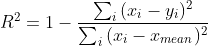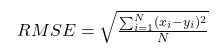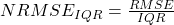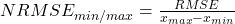# RECHARGE BUDDY

## Groundwater Recharge Estimation Tool

### Online Tool

→ 2. Background
→ 3. User Guide
→ 3.1. Quick start
→ 3.2. Home Module
→ 3.3. Input Data Module
→ 3.4. Analysis Module
→ 3.5. Output Timeseries
→ 3.6. Calibration Procedure
→ 3.7. Data inspection, visualisation and export
→ 4. Limitations
→ 6. References
→ 7. Contact

• ### 2. Background

##### The Water Table Fluctuation (WTF) method, widely used in hydrogeological studies (e.g., Healy and Cook, 2002; Scanlon and Healy, 2002; Coes et al., 2007; Labrecque et al., 2010) allows for estimation of groundwater recharge in unconfined aquifers based on the specific yield of the aquifer (or material) and the changes in water table elevation. Groundwater recharge is calculated using the following equation:• ### 3. User Guide

#### 3.3. Input Data Module

##### The number of columns, data format and the units of the various timeseries required for the input file are shown in the table below.
Columns Units Values RECHARGE BUDDY Input data file: format, columns, and units for input and user calibration data (UCD) DATE WTELEV UCD (max. 3 columns) yyyy-mm-dd m user choice eg. 2021-12-24 -1000 to 8000 user choice

#### 3.5. Output Timeseries

##### The output from RECHARGE BUDDY is shown in the table below.
 NAME DESCRIPTION Index Indicates the position of a specific record (line) in the timeseries. Change in WT Elev. (mm) Change in Water Table (WT) Elevation. For daily timestep the values in this column are calculated as the difference between Water Table (WT) Elevations for two consecutive days (i.e. ΔWT Elev. = WT Elevt+1 - WT Elevt). For all other averaging intervals this is calculated as the difference between the last day and the first day in the interval (e.g., for monthly this is calculated as the difference between the water table elevation on the last day of the month and the water table elevation on the first day of the month). The units for ΔWT Elev. are mm. Groundwater Recharge (mm) This is the amount of groundwater recharge and is always calculated using the daily timestep. When the data is displayed using averaging intervals other than daily, this is calculated as the sum of daily recharges (i.e., recharge is calculated only for the days when ΔWT Elev. is positive). The units for Groundwater Recharge are mm. To obtain the volume of recharge for a given area the user has to multiply this value with the land area of interest. Groundwater Discharge (mm) This is the amount of groundwater discharge and is always calculated using the daily timestep. When the data is displayed using averaging intervals other than daily, this is calculated as the sum of daily discharges (i.e., discharge is calculated only for the days when ΔWT Elev. is negative). The units for Groundwater Discharge are mm. To obtain the volume of discharge for a given area the user has to multiply this value with the land area of interest. Change in aquifer storage (mm) The change in aquifer storage is calculated on a daily basis as the product of ΔWT Elev. and specific yield. When positive, this indicates occurrence (i.e., dominance) of groundwater recharge, and when negative occurrence (i.e., dominance) of groundwater discharge. When the data is displayed using averaging intervals other than daily, this is calculated as the sum of daily changes, and thus, it shows the "net" change in storage over the respective averaging interval. The values calculated in this column are assigned to the Groundwater Recharge if positive and to Groundwater Discharge columns if negative. Consult sections 2 and 4 for additional details.

#### 3.6. Calibration Procedure

##### To aid with data inspection and assessment of the fitness of the output data, RECHARGE BUDDY includes several univariate and bivariate statistics. Univariate statistics, including, average, minimum, maximum and standard deviation are calculated separately for the input (i.e., user provided calibration data) and tool output time series. The graphs and the univariate statistics can be used for example for comparing the general trends, the range of values and the amplitude of variations in both data sets. The bivariate statistics include the coefficient of determination (R2), root mean square error (RMSE) and the normalized root mean square error (NRMSE). NRMSE is calculated by using the average, the interquartile range or the differences between maximum and minimum (see definitions below). The bivariate statistics are used for evaluating the fitness of the output, by providing a measure of the differences between the values calculated by the tool and the user provided calibration data (i.e., UCD). The equations used for calculating each bivariate statistic are shown below.##### Eq 2. - Coefficient of determination
###### R2 - coefficient of determination xi - value for observed data on day i yi - value for modelled data on day i xmean - mean of observed data##### Eq 3. - Root mean square error
###### N - number non-missing data points xi - value for observed data on day i yi - value for modelled data on day i##### Eq 4. - Normalized root mean square error average
###### NRMSEave - normalized root mean square error calculated using the average value of measured data RMSE - root mean square error xa - average value of observed data##### Eq 5. - Normalized root mean square error IQR
###### NRMSEIQR - normalized root mean square error calculated using minimum and maximum values of measured data RMSE - root mean square error IQR - interquartile range of the observed data; IQR = Q3-Q1,with Q3 = CDF-1(0.25), Q1 = CDF-1(0.75),where CDF is the quantile function